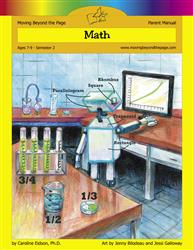# Common Core Alignment

CCSS.Math.Content.3.MD.7.a - Find the area of a rectangle with whole-number side lengths by tiling it, and show that the area is the same as would be found by multiplying the side lengths.

## 5: MathUnit 5: Area and Perimeter
Lesson 7: Calculating Area